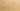# OutsideInMethodNesting

Misconception:

When nesting method calls, like with `a(b())`, calls are invoked from the outside in: first `a()` is invoked, then `b()` is invoked.Incorrect

Nested method calls are invoked outside in

Correct

Nested method calls are invoked inside out

## CorrectionHere is what's right.

The correct way to execute nested method calls is inside out. For example, when evaluating the expression `a(b())`, first `b()` is called, producing a value, then `a()` is called, receiving the return value of `b()` as an argument.

The corresponding expression tree looks as follows. First `b()` is called, returning a value of type `T`, and then `a()` is called with this value as an argument.

Note that for this composition of the two methods to work, the return type of `b()` needs to be compatible with the parameter type of `a()`.

The fact that the call `a()` needs the return value of `b()` as an argument implies that `b()` has to be called before `a()`, and thus that these calls evaluate from the inside out.

## SymptomsHow do you know your students might have this misconception?

This misconception occurred quite regularly, even for strong students. Here are two diagnostic probes to detect it.

### Expression Tree

Ask students to draw a tree for the expression `a(b())`. Students with this misconception may draw the following incorrect tree, where the inner piece of the expression—`b()`— is drawn as the root:

### Call Trace

Ask student to produce a call trace for code including the following statement:

``compute(left.eval(), right.eval());``

Students with this misconception may produce the following incorrect trace:

``````CALL compute
RETURN compute
CALL eval
RETURN eval
CALL eval
RETURN eval``````

Java